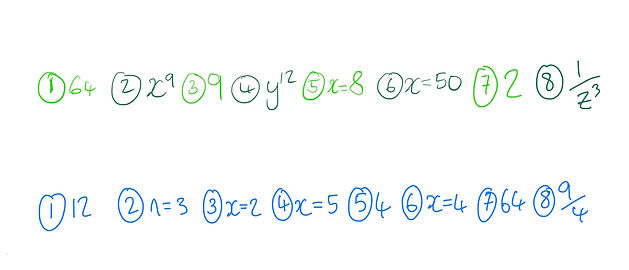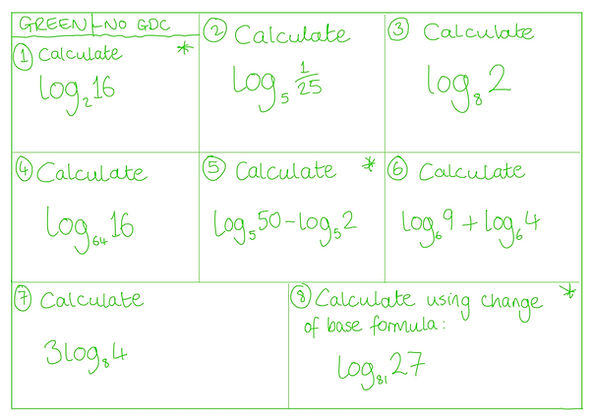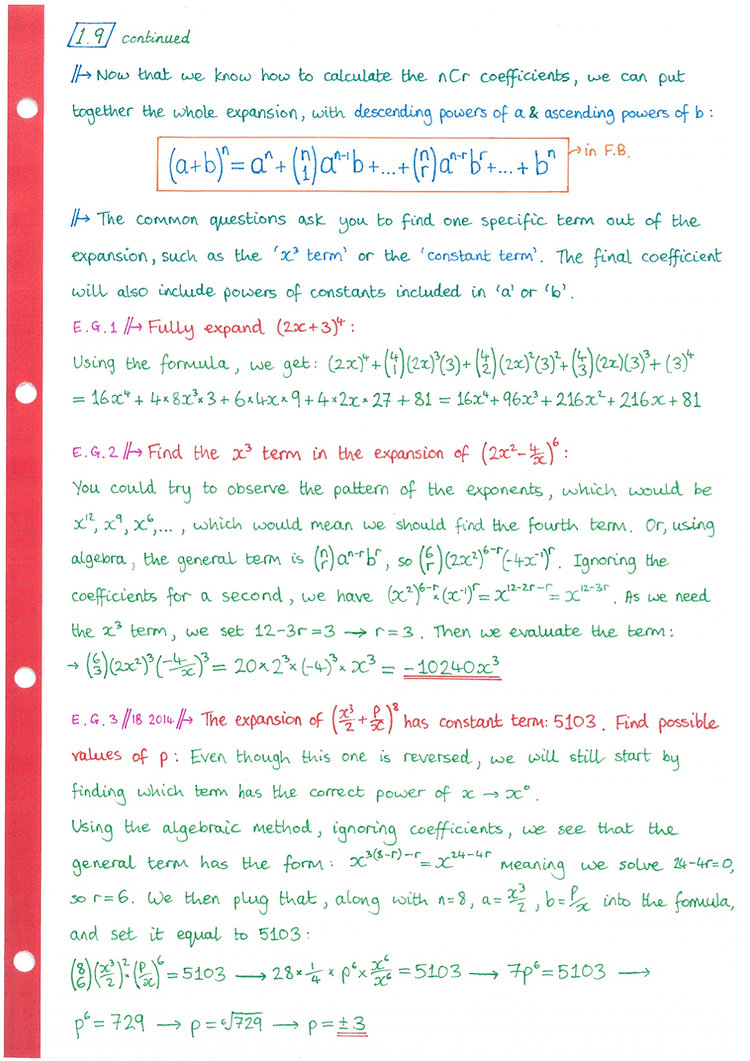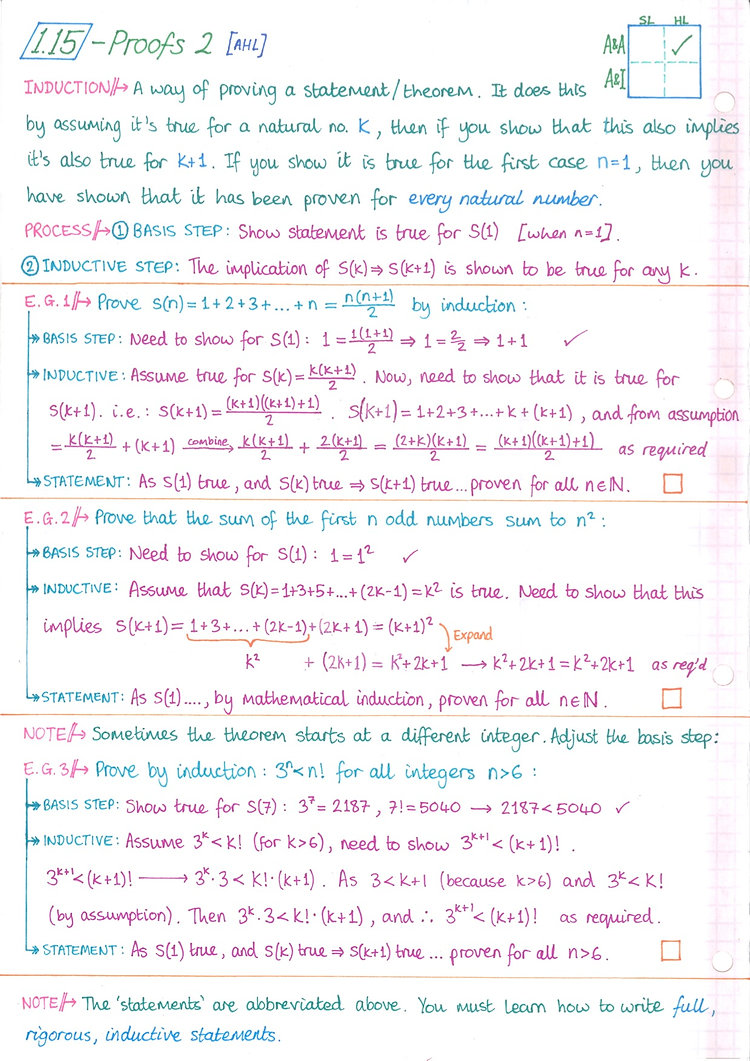1.1

1.2

1.3

1.4

1.5

1.6

1.7

1.8

1.9

1.10

1.11

1.12

1.13

1.14

1.15

1.16

1.1: Scientific notation1.2: Arithmetic SequencesExercisesSolutions1.3: Geometric Sequences1.4: Compound Interest1.5: Exponents & LogsExercisesSolutions1.6: Simple proofs1.7: More logarithmsExercisesSolutions1.8: Infinite Geometric Series1.9: Binomial TheoremExercisesSolutions1.10: Combinations & Permutations [AHL]1.11: Partial Fractions [AHL]1.12: Complex Numbers Intro [AHL]Videos1.13: Polar form & Euler's form [AHL]1.14: Complex roots & de Moivre's thm. [AHL]1.15: Proof by Induction, Contradiction, etc [AHL]1.16: Systems of 3 Equations [AHL]